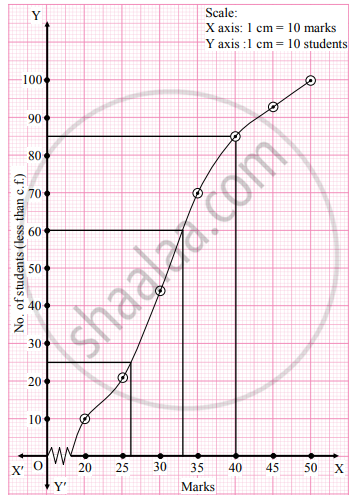# The following table gives frequency distribution of marks of 100 students in an examination. Marks 15 –20 20 – 25 25 – 30 30 –35 35 – 40 40 – 45 45 – 50 No. of students 9 12 23 31 10 8 - Mathematics and Statistics

Graph

The following table gives frequency distribution of marks of 100 students in an examination.

 Marks 15 –20 20 – 25 25 – 30 30 –35 35 –  40 40 – 45 45 – 50 No. of students 9 12 23 31 10 8 7

Determine D6, Q1, and P85 graphically.

#### Solution

To draw an ogive curve, we construct the less than cumulative frequency table as given below:

 Marks No. of students (f) Less than cumulative Frequency(c.f.) 15 – 20 9 9 20 – 25 12 21 25 – 30 23 44 30 – 35 31 75 35 – 40 10 85 40 – 45 8 93 45 – 50 7 100 Total 100

The points to be plotted for less than ogive are (20, 9), (25, 21), (30 , 44), (35, 75), (40, 85), (45, 93), (50, 100).Here, N = 100
For D6, (6"N")/(10) = (6(100))/(10) = 60

For Q1, "N"/4=100/4 = 25

For P85, (85"N")/100=(85xx100)/100 = 85

∴ We take the points having Y coordinates 60, 25, and 85 on Y-axis. From these points, we draw lines parallel to X-axis. From the points where these lines intersect the curve, we draw perpendiculars on X-axis.
X coordinates of these points give the values of D6, Q1, and P85.

∴ D6 = 32.5, Q1 = 26, P85 = 40

Concept: Graphical Location of Partition Values
Is there an error in this question or solution?
Chapter 1: Partition Values - Exercise 1.3 [Page 18]

Share